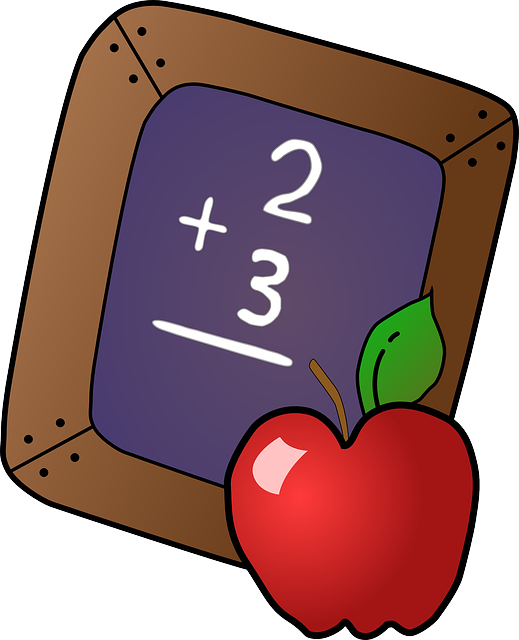MATHAddition Add 'Em Up - (primarygames.com) - add two numbers and click on correct answer Addition Concentration - (aplusmath.com) - two-digit addition - fun (needs JAVA) Addition Flashcards - (aplusmath.com) - great easy math Addition Hidden Picture - (aplusmath.com) - fun math (needs JAVA) Addition Matho - (aplusmath.com) - fun game - two-digit addition (needs JAVA) Addition Mystery Game - (dositey.com) - great Addition Surprise! - (harcourtschool.com) Addition Tunnel Blaster - (playkidsgames.com) - Make sure to read the instructions - keep on your toes for this activity Spacey Math - (learningplanet.com) - addition, subtraction, multiplication and division games - fun The Number Monster - (coolmath4kids.com) - addition, subtraction, multiplication, division - levels from easy to killer - good Two Minute Warning - (primarygames.com) - two minutes to do fairly simple math (0-19) (add, subtract, multiplication, and division) Counting Count Us In - Games to help students understand basic number concepts Decimals Decimal and Fraction matching - (oswego.org) Division Division Facts - (quia.com) - 2 game choices - good Spacey Math - (learningplanet.com) - addition, subtraction, multiplication and division games - fun The Number Monster - (coolmath4kids.com) - addition, subtraction, multiplication, division - levels from easy to killer - good Two Minute Warning - (primarygames.com) - two minutes to do fairly simple math (0-19) (add, subtract, multiplication, and division) Fractions Decimal and Fraction matching - (oswego.org) Equivalent Fractions - (quia.com) - 3 game choices - good Fresh Baked Fractions - FunBrain Who Wants Pizza? - (math.rice.edu) - activity about Fractions - fun and simple Geometry Geometric Terms - (quia.com) - 4 games choices - good for upper grades Graphing and Line Plots Billy Bug - (oswego.org) - grid interactive game Measurement (km, m, cm, kg, g, lb, oz, l, ml, hr, min, sec) Customary Measurement - (quia.com) - 3 game choices - good for upper grades Money Change Maker - FunBrain Counting Change - (quia.com) - 3 game choices - good Multiplication Multiplication Facts Review - (quia.com) - 3 game choices - good Multiplication Mystery - (harcourtschool.com) - like "Addition Surprise" only it's multiplication Spacey Math - (learningplanet.com) - addition, subtraction, multiplication and division games - fun The Number Monster - (coolmath4kids.com) - addition, subtraction, multiplication, division - levels from easy to killer - good Two Minute Warning - (primarygames.com) - two minutes to do fairly simple math (0-19) (add, subtract, multiplication, and division) Order (putting in order) Ordering : Least to Greatest - (oswego.org) Patterns Pattern Blocks and Manipulatives - (arcytech.org) - online activity Place Values AAA Math - 3rd Grade - (aaamath.com) - click on Place Values (there are several) - games are below instructions Place Value Game - (funbrain.com) - Funbrain Place Value Placer - FunBrain Subtraction Spacey Math - (learningplanet.com) - addition, subtraction, multiplication and division games - fun Subtraction Tunnel Blaster - (playkidsgames.com) - includes negative numbers Take it Away - (primarygames.com - subtract two numbers and click on correct answer The Number Monster - (coolmath4kids.com) - addition, subtraction, multiplication, division - levels from easy to killer - good Two Minute Warning - (primarygames.com) - two minutes to do fairly simple math (0-19) (add, subtract, multiplication, and division) Telling Time Willy the Watchdog - (harcourtschool.com) - telling time Tick Tock... on the Office Clock - great links to worksheets and games for Telling Time skills General Math Sites - variety of activities Easy Roman Numerals - (quia.com) - 4 game choices -- good Fifth Grade Math - (aaamath.com) - excellent site FunBrain - lots of games (Math) One False Move Math Baseball - two players Change Maker Fresh Baked Fractions Cookie Dough Place Value Placer Tic Tac Toe Squares Harcourt Math Activities - (need Shockwave) - free preview activities from the textbook website Internet4classrooms -- great site - scroll down to the bottom for various grades --Assessment category Leap for Fun - interactive board game for 1-4 players (needs Shockwave) Learning Planet - several online math games (Math Mayhem, Fraction Frenzy, Spacey Math) Math links - (dositey.com) - click on the appropriate grade level Math Online - (mhschool.com) - preview activities from the textbook by grade Mathematics Index - (kidport.com) - click on the appropriate problems - easy to harder Riley's Monster Crunch - (kidscom.com) - like a computer game program - really good Room 108 Math - (netrover.com) - great links to some fun games The Little Animals Activity Center - (bbc.co.uk) - cute site with activities including math and reading More Math Sites: Needs Some Preparation Dositey.com - free interactive lessons, exercises and worksheets, educational games, open-ended questions in Math and Language Arts by grades (explore which games are ready to use before hand) Math Online - (mhschool.com) - textbook activities - choose grade Harder Math - Teachers can check out this website to see if it will help you - Teacher Resource Interactive Examples of NCTM Standards - (standards.nctm.org) - good for all grades - patterns and grids